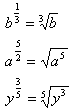Introduction

As discussed in the last book, another way to write a fractional exponent is with a radical sign. The examples below demonstrate how to translate a fractional exponent into a radical expression:In cases where there are many terms under the radical sign it may be easier to simplify using roots rather than translating the expression to fractional exponents.

Listen